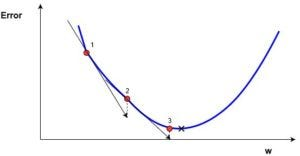First of all I just want to put this out as a disclaimer; I’m new to both machine learning and pytorch, and my calculus is limited to single variable. With that said, I’m trying to get some intuition about how, why and when gradients are used in neural networks, as they seem to play an important role. From what I’ve learned so far, a gradient is basically the derivative of a vector. I also understand that the chain rule is applied at some point in the process. However, my knowledge of the gradient and its application in a neural network pretty much ends there.

Now let’s get to my questions. Let’s start with the “when” (or rather “where” in this case). Where in the network are the gradients utilized? I’m fairly certain they play a role in back propagation, as I noticed playing around with pytorch that calling the backward() function of a tensor in pytorch changes its .grad value. Are gradients used somewhere else in the neural network, aside from back propagation?

Secondly, what exactly is the purpose of the gradient, and how is it utilized on an intuitive level? Right now, I’m thinking of the neural network and its components in terms of plain numbers, so it’s hard to imagine finding anything to “take the derivative of” or use the chain rule on since I don’t see a function anywhere. Or should I think of the entire graph as just one giant function? If so, what valuable data do we get from “taking the gradient” (if that’s the proper expression)?

Finally, I’ve played around with pytorch quite a bit the last few weeks. As I mentioned above, used the backward() function on a tensor which updated its .grad values. However, its new .grad values seemed to be completely unrelated to its original values. What’s going on here?

I’m not sure how much sense these questions will make. Anyway, I appreciate any help I can get.

Thanks!

I’ll try to write a very simple explanation to get you an intuition about neural networks and gradients in general.
There are a lot of books, courses etc. that most likely introduce much better to the topic.

Imagine we would like to “find” the best weights for the function `f(x) = w * x`, where we already have some data (`x`) and targets (`f(x) = y`). Let’s just assume the ground truth value of our parameter `w` is `3`. In a real use case we wouldn’t know the best values for the parameter, but let’s cheat a bit for this example.
Using the function, we can create a model and just initialize its `w` to a random number.
We end up with `w = 1` as our initial value. This step is referred to as weight initialization.

Now we can calculate the model output using our initial `w` and compare it to the target we already have. To get a meaningful value of the discrepancy we use a loss function to measure our error. You can imaging the loss function creates a “loss surface”, where for each parameter value, we have a specific loss value.
Let’s just assume our loss function looks like this:Using our initial value for `w` we end up at position 1.

Since our `w` is a bit too low, we would like to increase it a bit.
So let’s use the derivative (gradient) at the current position to update w.
w = w - learning_rate * dL/dw
Because dL/dw is negative, the new value for w will be bigger than before. In the end we move towards the optimum and end up after the weight update at position 2 in the image.
Because sometimes the derivative is quite large, we could also jump that far, that we miss the optimum and end up on the other side of the loss function (right of the optimum).
This is why we scale the derivative with a learning rate to scale the weight updates. The closer you are to your optimum, the lower your learning rate should be. This methodology is implemented in e.g. `ReduceLROnPlateau` or other learning rate schedulers.

This is the basic approach for neural networks. The big difference is, that we have a lot of parameters (sometimes millions), which are connected by non-linearities. To calculate the gradient of the loss with respect to all parameters, we can use the back propagation.
The loss surface is also dependent on the parameters and thus high-dimensional.
In the simple example we just used one weight, which created this nice looking loss function.
In reality we would have something like a 1,000,000-dimensional loss surface, which is quite hard to imaging.

I hope it makes things a bit clearer. I’ve heard a some good things about the fast.ai course, so maybe you should check it out. I’m sure they introduce the topic much better.4 Likes

speculating => reduced echelon form for y with regression and gradient input on multiple matrix reversal for new x conditional - Oh, b check’n into that fast.ai

thx ed

1 Like

Thank you! It did clear up a few things, but I still feel clueless about how to think of gradients when using pytorch. For example, how do I know if I should set requires_grad to True or False and things like that. I will check out fast.ai and return with further questions if I have any (or maybe ask them over there, since their forum is aimed mainly towards beginners).

If you are just working with “vanilla” neural networks, i.e. you just use the `nn.Modules`, you usually don’t need to set `requires_grad` anywhere.
You would have to define your model, data, criterion, and are good to go.
Also, have a look at the PyTorch Tutorials.

Wow this is extremely helpful and will also check out the PyTorch tutorials also, meanwhile i have been learning some good stuff from concept explainers about z-scores and multivariable functions. It is a beginner level info but good to follow.

1 Like